# SAT II Math I : Maximum and Minimum

## Example Questions

### Example Question #41 : Functions And Graphs

What is the vertex of the following function? Is it a maximum or a minimum?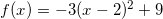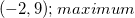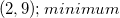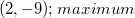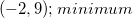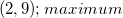Explanation:

The equation of a parabola can be written in vertex form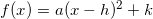where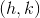is the vertex anddetermines if it is a minimum or maximum. Ifis positive, then it is a minimum; ifis negative, then it is a maximum.In this example,is negative, so the vertex is a maximum.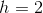and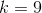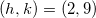### Example Question #42 : Functions And Graphs

Determine the maximum or minimum of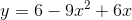.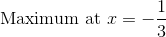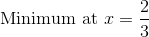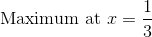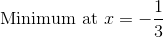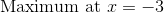Explanation:

Rewrite the equation by the order of powers.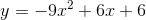This is a parabola in standard form: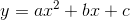Determine the values of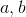to the vertex formula.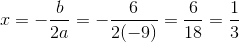Since the leading coefficient of the parabola is negative, the parabola will curve downward and will have a maximum point.

Therefore there is a maximum at,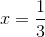.